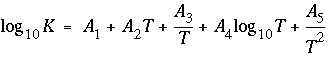| Next|| Previous || Top |

# PHASES

This keyword data block is used to define a name, chemical reaction, log K , and temperature dependence of log K for each gas component and mineral that is used for speciation, batch-reaction, transport, or inverse-modeling calculations. Normally, this data block is included in the database file and only additions and modifications are included in the input file.

### Example data block

```Line 0:  PHASES
Line 1a: Gypsum
Line 2a:      CaSO4:2H2O = Ca+2 + SO4-2 + 2H2O
Line 3a:      log_k     -4.58
Line 4a:      delta_h   -0.109
Line 5:       -analytical_expression 68.2401 0.0 -3221.51  -25.0627  0.0
Line 1b: O2(g)
Line 2b:      O2 = O2
Line 3b:      log_k     -2.96
Line 4b:      delta_h   1.844```

### Explanation

Line 0: PHASES

Keyword for the data block. No other data are input on the keyword line.

Line 1: Phase name

phase name --Alphanumeric name of phase, no spaces are allowed.

Line 2: Dissolution reaction

Dissolution reaction for phase to aqueous species. Any aqueous species, including e - , may be used in the dissolution reaction. The chemical formula for the defined phase must be the first chemical formula on the left-hand side of the equation. The dissolution reaction must precede any identifiers related to the phase. The stoichiometric coefficient for the phase in the chemical reaction must be 1.0.

Line 3: log_k log K

log_k--Identifier for log K at 25 o C. Optionally, -log_k, logk, -l[ og_k], or -l[ ogk].

log K --Log K at 25 o C for the reaction. Default is 0.0.

Line 4: delta_h enthalpy, [ units ]

delta_h--Identifier for enthalpy of reaction at 25 o C. Optionally, -delta_h, deltah, -d[ elta_h], or -d[ eltah].

enthalpy --Enthalpy of reaction at 25 o C for the reaction. Default is 0.0.

units --Units may be calories, kilocalories, joules, or kilojoules per mole. Only the energy unit is needed (per mole is implied) and abbreviations of these units are acceptable. Explicit definition of units for all enthalpy values is recommended. The enthalpy of reaction is used in the van't Hoff equation to determine the temperature dependence of the equilibrium constant. Internally, all enthalpy calculations are performed in the units of kilojoules per mole. Default units are kilojoules per mole.

Line 5: -analytical_expression A 1 , A 2 , A 3 , A 4 , A 5

-analytical_expression--Identifier for coefficients for an analytical expression for the temperature dependence of log K . If defined, the analytical expression takes precedence over the van't Hoff equation to determine the temperature dependence of the equilibrium constant. Optionally, analytical_expression, a_e, ae, -a[ nalytical_expression], -a[ _e], -a[ e].

A 1 , A 2 , A 3 , A 4 , A 5 --Five values defining log K as a function of temperature in the expression, where T is in Kelvin.

### Notes

The set of lines 1 and 2 must be entered in order, either line 3 ( log_k) or 5 ( -analytical_expression) must be entered for each phase. The analytical expression ( -analytical_expression) takes precedence over the van't Hoff equation ( delta_H) to determine the temperature dependence of the equilibrium constant. Lines 3, 4, and 5 may be entered as needed in any order. Additional sets of lines 1 through 5 may be added as necessary to define all minerals and gases. The equations for the phases may be written in terms of any aqueous chemical species, including e - .

The identifiers -no_check can be used to disable checking charge and elemental balances (see SOLUTION_SPECIES). The use of -no_check is not recommended, except in cases where the phase is only to be used for inverse modeling. Even in this case, equations defining phases should be charge balanced. The identifier can also be used to define the mineral formula for an exchanger with an explicit charge imbalance (see explanation under EXCHANGE).

### Example problems

The keyword PHASES is used in example problems 1, 6, 8, 9, 10, 16, and 18.

### Related keywords

EQUILIBRIUM_PHASES, EXCHANGE, INVERSE_MODELING, KINETICS, REACTION, SAVE equilibrium_phases, and USE equilibrium_phases.

| Next|| Previous || Top |